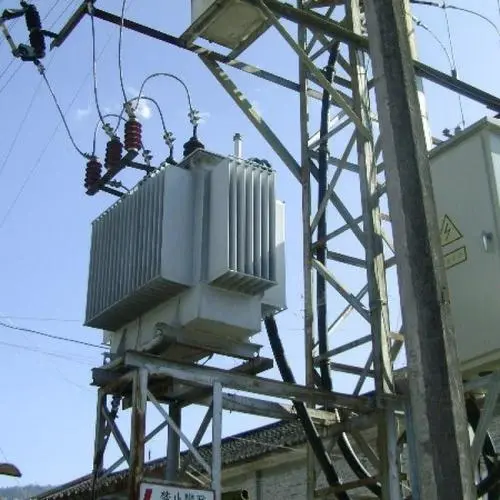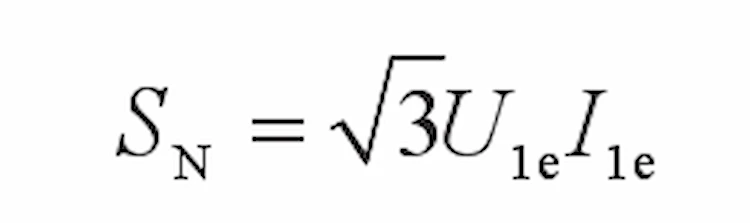# How to Determine the Size of the Rated Capacity of the Transformer?After the power transformer is overhauled or handed over, various electrical tests can be carried out according to the data on the nameplate.

Under the condition of test conditions, such as three-phase AC power supply, transformer, wattmeter, etc. on site, the no-load test of the transformer can be carried out, and the rated capacity of the transformer can be calculated according to the no-load test results.

However, when there is no three-phase AC voltage and test equipment at the construction site, it is difficult to determine the rated capacity of the transformer without a nameplate.

A method is summed up from the field work practice, which can identify the rated capacity of the transformer that does not have the test conditions and has no nameplate on site, and further calculate other data on the transformer nameplate. The operation steps are as follows:

(1) Remove all loads on the low-voltage side of the transformer, disconnect the circuit breaker on the high-voltage side of the transformer or open the drop switch on the high-voltage side; open the isolating switch or knife switch on the low-voltage side to keep the transformer in a power outage state.

(2) Adjust the clamp-type ammeter to the AC current block, and clamp the clamp-type ammeter to any one of the phases of the high-voltage lead of the high-voltage side of the transformer. After that, close the circuit breaker or drop switch on the high-voltage side of the transformer to apply the rated voltage to the high-voltage side of the transformer. At this time, the low-voltage side of the transformer is in an open-circuit state. Side no-load current I0.

(3) Generally, the no-load current of small capacity is 2% to 6% of the rated current of the transformer, that is, I0≈(2% to 6%) I1e; the no-load current of large capacity is 0.6% to 1.8% of the rated current of the transformer, that is, I0 ≈(0.6% to 1.8%) I1e.

(4) When the transformer is S series, the no-load current is about 2% of the rated current of the transformer, that is, I0≈ 2%I1e. According to the no-load current I0 value recorded by the clamp-type ammeter, the rated current I1e of the high-voltage side of the transformer can be calculated.

Then calculate the rated capacity of the transformer according to the formula:In the formula, U1e——the rated voltage value of the high voltage side of the transformer, kV;
I1e——The rated current value of the high voltage side of the transformer, A;
SN—transformer rated capacity, k V · A.
I1e can be obtained by calculation, U1e is known, and the rated capacity of the transformer can be calculated by substituting the values of U1e and I1e into the above formula.

##### Read more about the Ultimate 35kv Class Power Distribution Transformer Guide

(5) When the transformer is of SJ series, the no-load current is about 3.5% of the rated current of the transformer, that is, I0≈3.5%I1e. According to the no-load current I0 value recorded by the clamp ammeter, the rated current I1e of the transformer can be calculated. Then calculate the rated capacity of the transformer according to the above formula for calculating the rated capacity of the transformer.

(6) The rated capacity value of the transformer without a nameplate can be determined according to the field test value; other rated parameters of the transformer can be calculated through the rated capacity. This test method can be used to identify the rated capacity of transformers without test conditions and without a license on site, and has been applied in field work for many times. The method is accurate and simple.

## Share:

### 10 kv Oil Filled Transformer

Fault Diagnosis and Analysis of 10 kv Oil Filled Transformer 10 kv Oil filled transformer is a key equipment for power distribution, and its failure